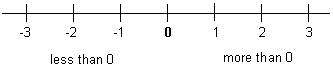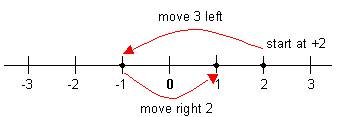# Directed Numbers Math Review

math tutorials > directed numbers

## Directed numbers are just numbers with a positive or negative sign in front of them!

Directed numbers are signed numbers i.e. numbers with a positive or plus sign (+) in front of them or a negative or minus sign (-) in front of them  e.g.  +6, -2, +7 etc.  All these whole numbers (and 0) grouped together are called integers.

These numbers can be represented on a number line.A positive number signifies a movement to the right.
A negative number signifies a movement to the left.

A number lacking a sign is positive.

e.g.  +2 + -3 + 2+2 + -3 + 2 = +1

Note:  A double negative changes to a single positive e.g.  +3 - -3 = +3 +3 = +6

A  negative sign with a positive sign changes to a single negative
e.g.  3 + -4 = 3 – 4 = -1
3 + -4 = 3 – 4 = -1

## Multiplying and Dividing.

The following rules apply:

 + x + = + +6 x +6 = +36 + x - = - +6 x -6 = -36 - x + = - -6 x +6 = -36 - x - = + -6 x -6 = +36

The same rules apply for division

## Math problems for you to try.

Note:  Remember the correct order of operations.

1. 8 - 11
2. 5 - (-2)
3. 9 - (6 - 9)
4. -3 x -4
5. -11 - (3 + 4)
6. 3 x (-2)²

Solutions:  1)  -3    2)  +7    3)  +12    4)  +12    5)  -18    6)  +12